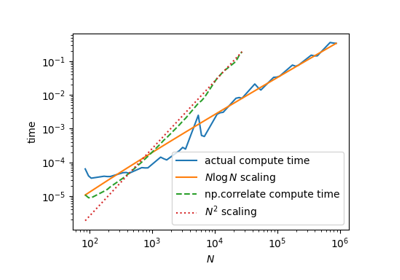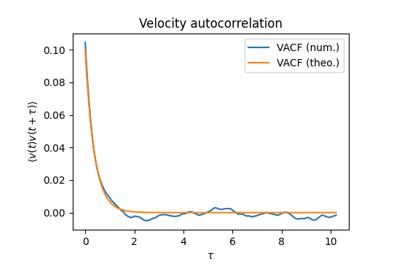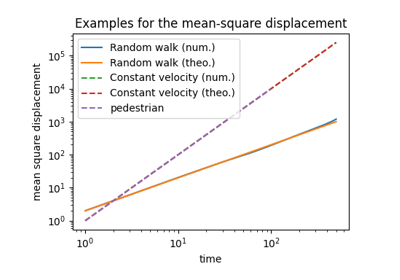# Examples of using tidynamics¶

The examples below show how one can use tidynamics to compute correlations and mean-squared displacements (MSD).

The example “Command-line interface to tidynamics” runs in the command-line. It performs the two basic operations of tidynamics (autocorrelation function and MSD) using files as input data. The file random_steps_sample_0.txt.gz contains example data for computing the autocorrelation function and the file random_walk_sample_0.txt.gz contains example data for computing the MSD.

The following commands run the command-line tool:

python tidynamics_tool.py msd random_walk_sample_0.txt.gz random_walk_msd_0.txt
python tidynamics_tool.py acf random_steps_sample_0.txt.gz random_steps_acf_0.txt


The output is stored in the files random_walk_msd_0.txt and random_steps_acf_0.txt.

The other examples require matplotlib.Command-line interface to tidynamicsScaling behaviourCorrelation functionsMean-square displacements (MSD)

Gallery generated by Sphinx-Gallery